Maths-
General
Easy

Question

# 𝐴𝑃 is perpendicular bisector of 𝐵𝐶. 1. What segment lengths are equal? Explain your reasoning. 2. Find AB.3. Explain why D is on 𝐴𝑃.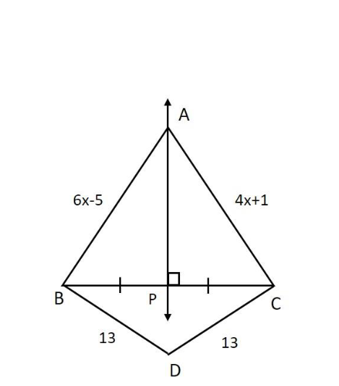Hint:

## The correct answer is: 1. equal segments AB = BC, BD = DC and BP = PC 2. AB = 4 units. 3.Yes, D lie on AP.

### Answer: Step by step explanation:  Given: AB = 6x - 5.AC = 4x + 1.BD = 13DC = 13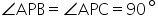AP is perpendicular bisector at BC. Step 1: In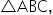AP is perpendicular bisector.Therefore, points on AP are equidistant from B and CSo,AB = AC6x – 5 = 4x + 16x – 4x = 5 + 12x = 6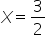x = 1.5AB = 6x – 5AB = 6(1.5) – 5AB = 9 – 5AB = 4 Step 2: Given: BD = 13DC = 13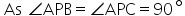Therefore,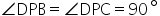Also, BP = PCDP is perpendicular bisector on BC from D.Also,As point D is equidistant from B and CTherefore, D lies on DP Final Answer:  equal segments AB = BC, BD = DC and BP = PC AB = 4 units. Yes, D lie on AP.#### With Turito Foundation.#### Get an Expert Advice From Turito.